Скачать презентацию Aims to inspire pupils confidence in mathematics

522fc45255470344bb920c0c939f3f48.ppt

• Количество слайдов: 28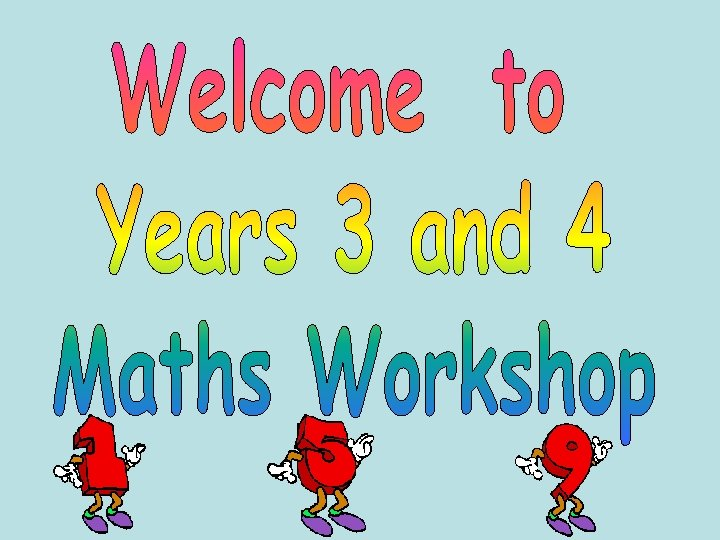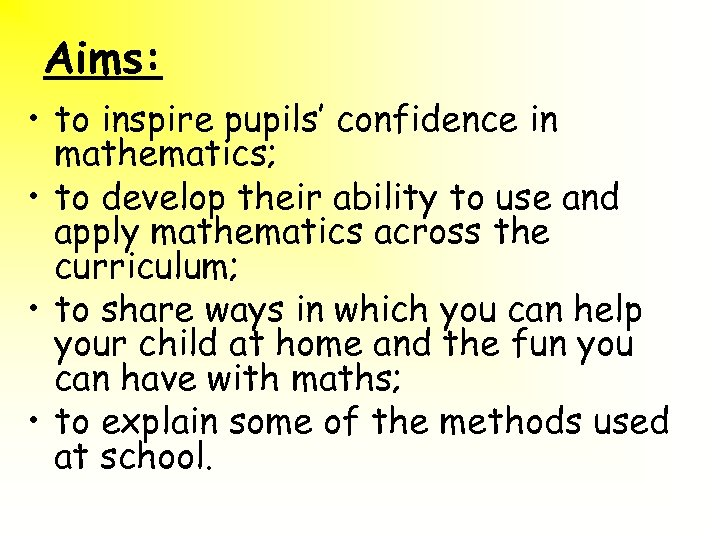Aims: • to inspire pupils’ confidence in mathematics; • to develop their ability to use and apply mathematics across the curriculum; • to share ways in which you can help your child at home and the fun you can have with maths; • to explain some of the methods used at school.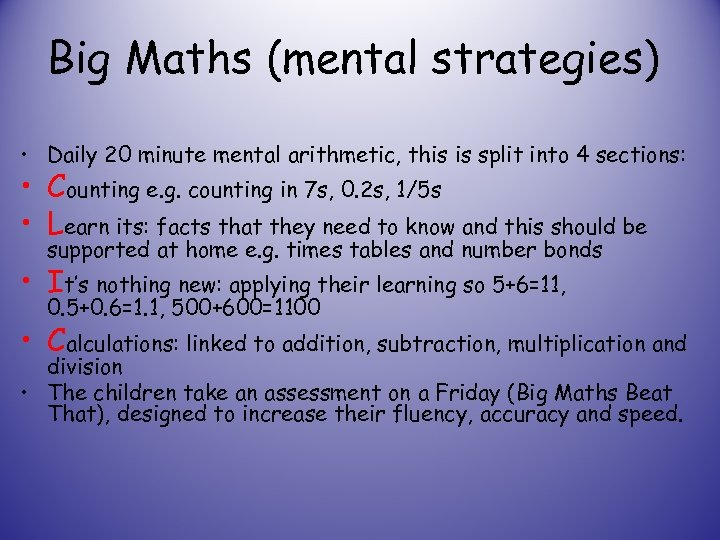Big Maths (mental strategies) • Daily 20 minute mental arithmetic, this is split into 4 sections: • Counting e. g. counting in 7 s, 0. 2 s, 1/5 s • Learn its: facts that they need to know and this should be supported at home e. g. times tables and number bonds • It’s nothing new: applying their learning so 5+6=11, 0. 5+0. 6=1. 1, 500+600=1100 • Calculations: linked to addition, subtraction, multiplication and division • The children take an assessment on a Friday (Big Maths Beat That), designed to increase their fluency, accuracy and speed.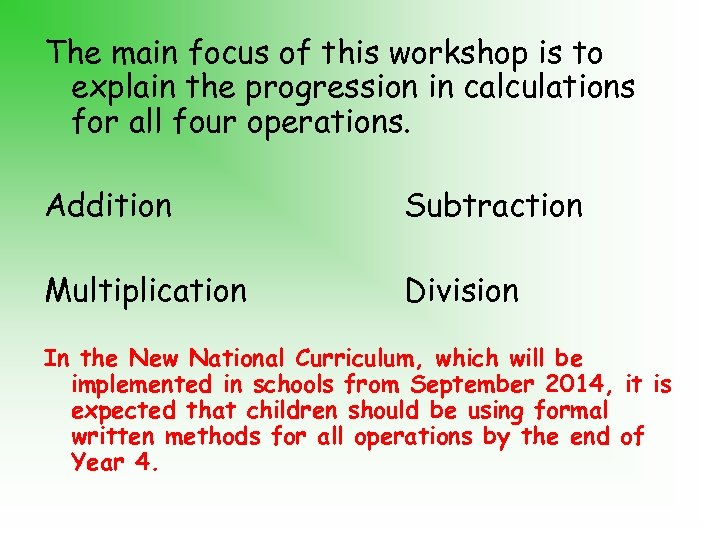The main focus of this workshop is to explain the progression in calculations for all four operations. Addition Subtraction Multiplication Division In the New National Curriculum, which will be implemented in schools from September 2014, it is expected that children should be using formal written methods for all operations by the end of Year 4.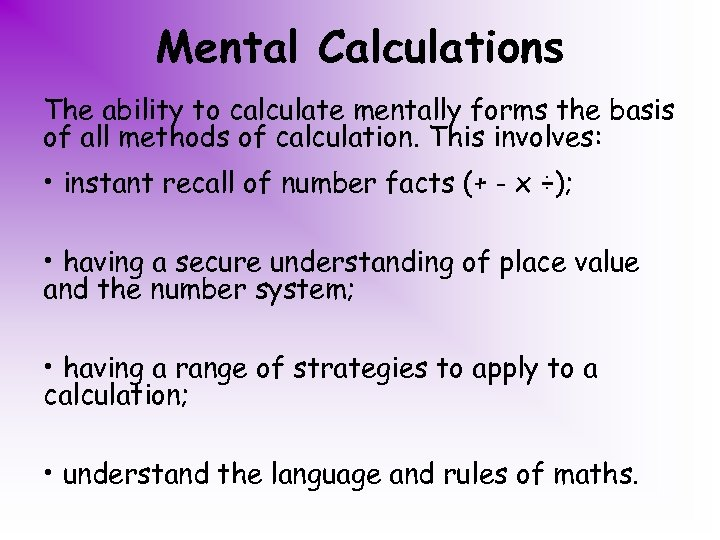Mental Calculations The ability to calculate mentally forms the basis of all methods of calculation. This involves: • instant recall of number facts (+ - x ÷); • having a secure understanding of place value and the number system; • having a range of strategies to apply to a calculation; • understand the language and rules of maths.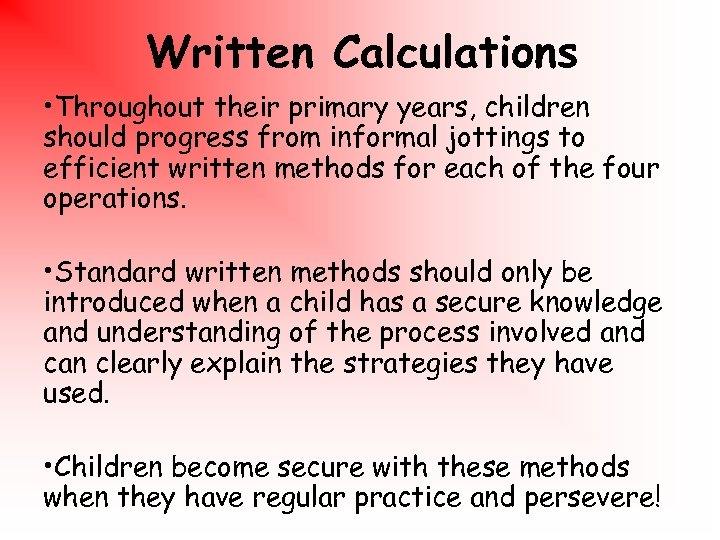Written Calculations • Throughout their primary years, children should progress from informal jottings to efficient written methods for each of the four operations. • Standard written methods should only be introduced when a child has a secure knowledge and understanding of the process involved and can clearly explain the strategies they have used. • Children become secure with these methods when they have regular practice and persevere!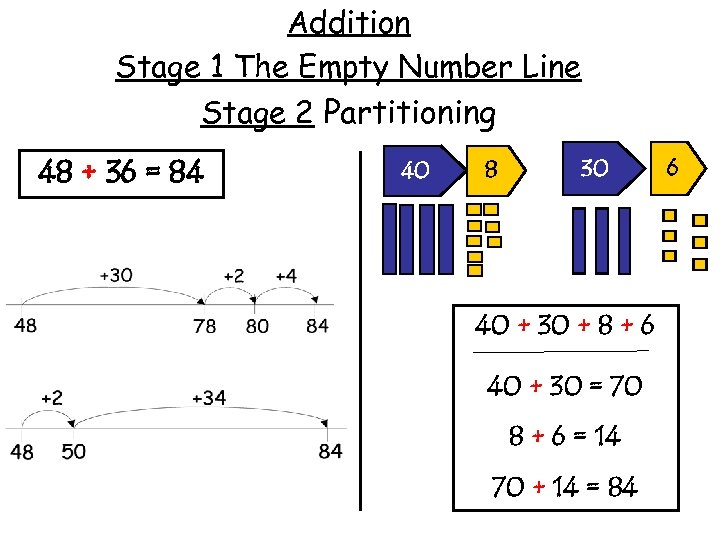Addition Stage 1 The Empty Number Line Stage 2 Partitioning 48 + 36 = 84 40 8 30 40 + 30 + 8 + 6 40 + 30 = 70 8 + 6 = 14 70 + 14 = 84 6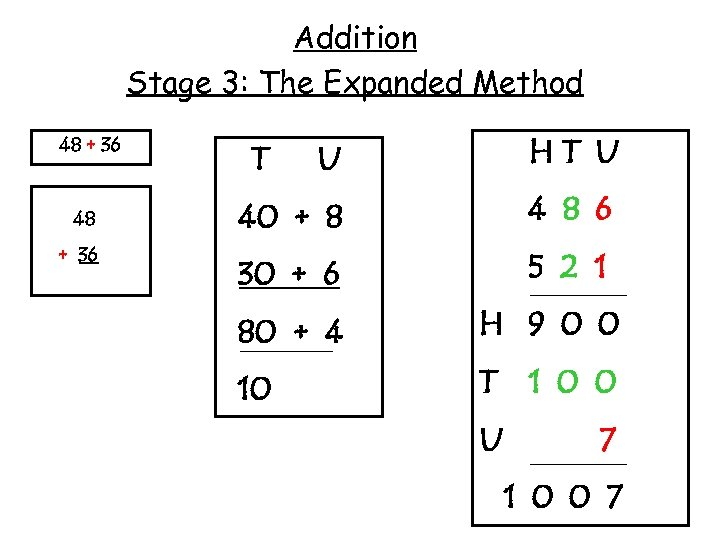Addition Stage 3: The Expanded Method 48 + 36 U HT U 40 + 8 4 8 6 30 + 6 5 2 1 T 80 + 4 H 9 0 0 10 T 1 0 0 U 7 1 0 0 7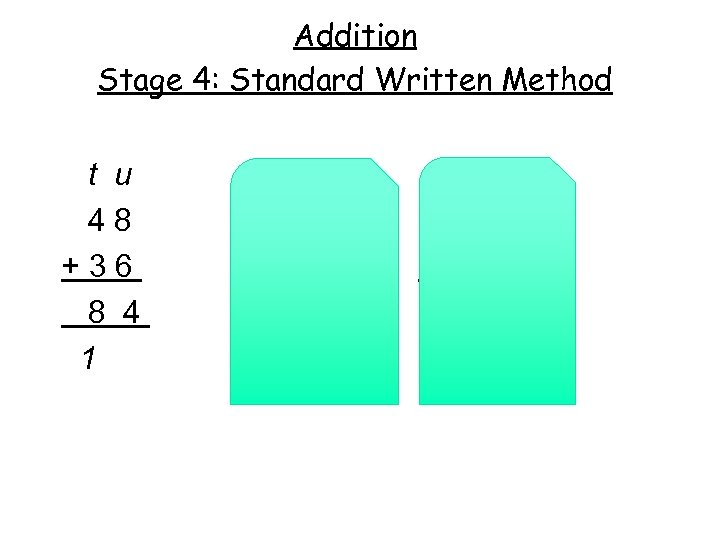Addition Stage 4: Standard Written Method t u 48 +36 8 4 1 htu 156 + 75 231 11 u. 1/10 3. 6 + 1. 6 5. 2 1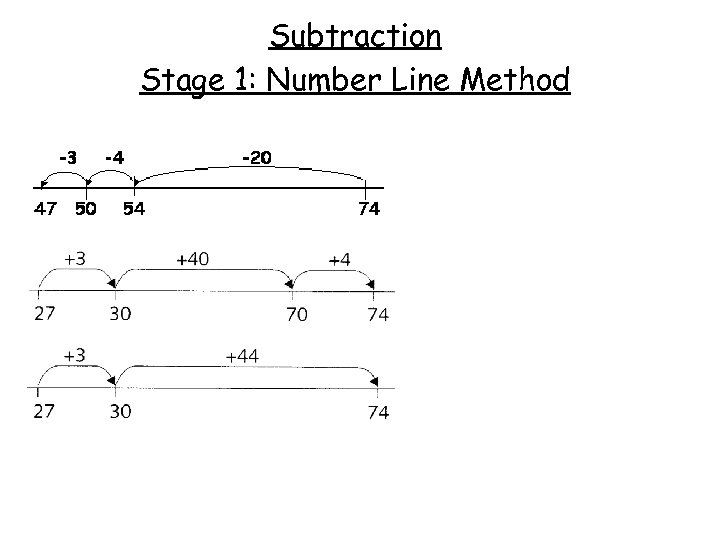Subtraction Stage 1: Number Line Method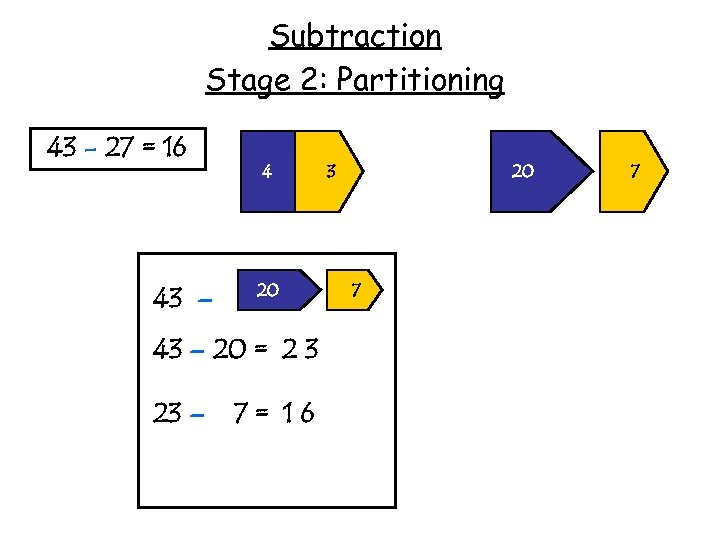Subtraction Stage 2: Partitioning 43 - 27 = 16 43 – 4 20 43 – 20 = 2 3 23 – 7= 16 3 20 7 7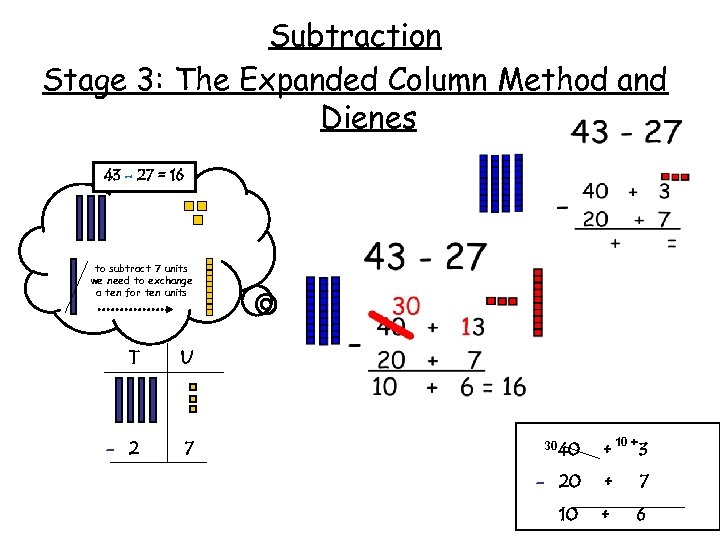Subtraction Stage 3: The Expanded Column Method and Dienes 43 - 27 = 16 to subtract 7 units we need to exchange a ten for ten units T U - 2 7 10 + 30 40 + 3 - 20 + 7 10 + 6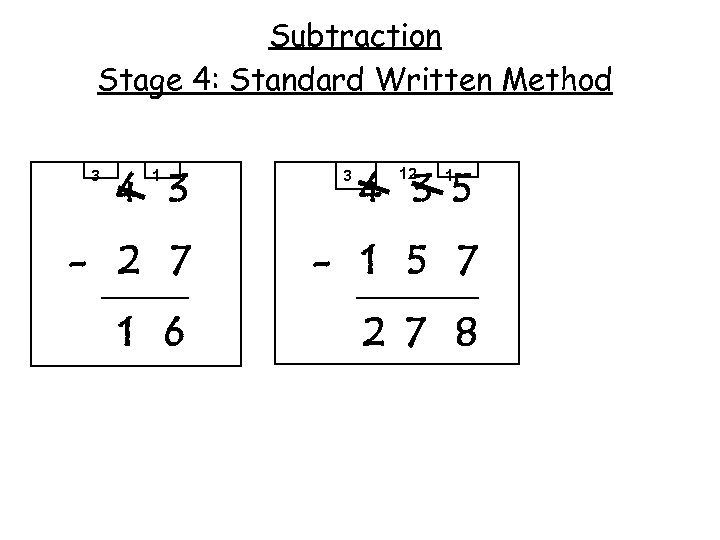Subtraction Stage 4: Standard Written Method 3 4 3 1 3 4 3 5 12 1 - 2 7 - 1 5 7 1 6 2 7 8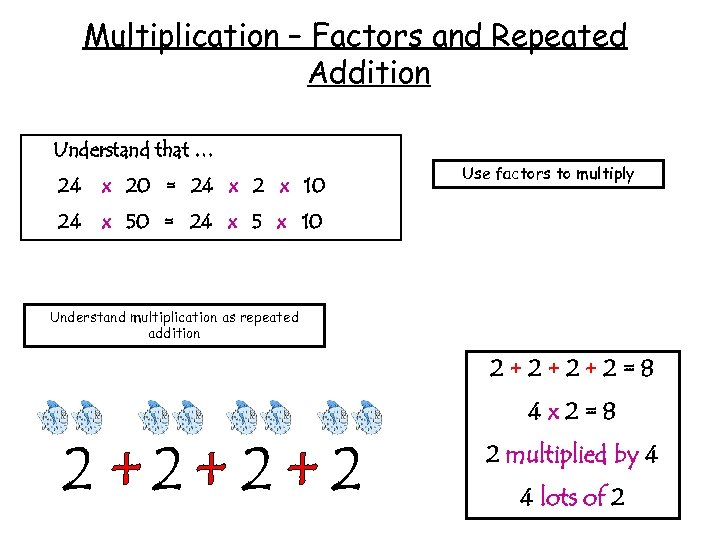Multiplication – Factors and Repeated Addition Understand that … 24 x 20 = 24 x 2 x 10 24 Use factors to multiply x 50 = 24 x 5 x 10 Understand multiplication as repeated addition 2+2+2+2=8 4 x 2=8 2 multiplied by 4 4 lots of 2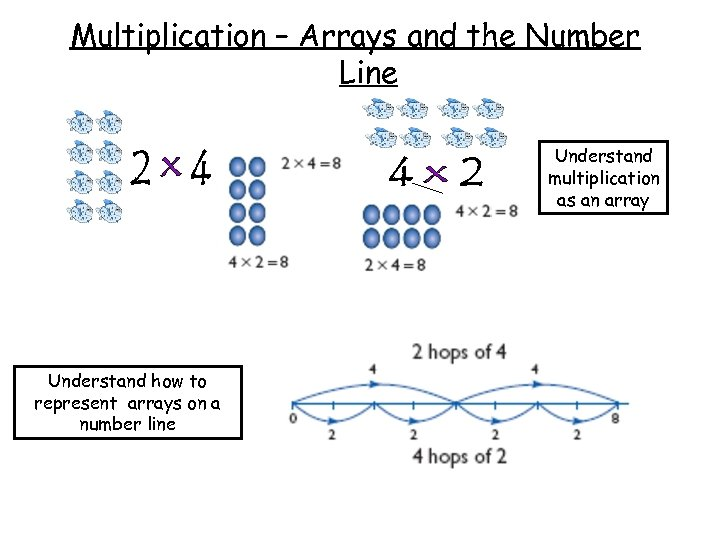Multiplication – Arrays and the Number Line Understand multiplication as an array Understand how to represent arrays on a number line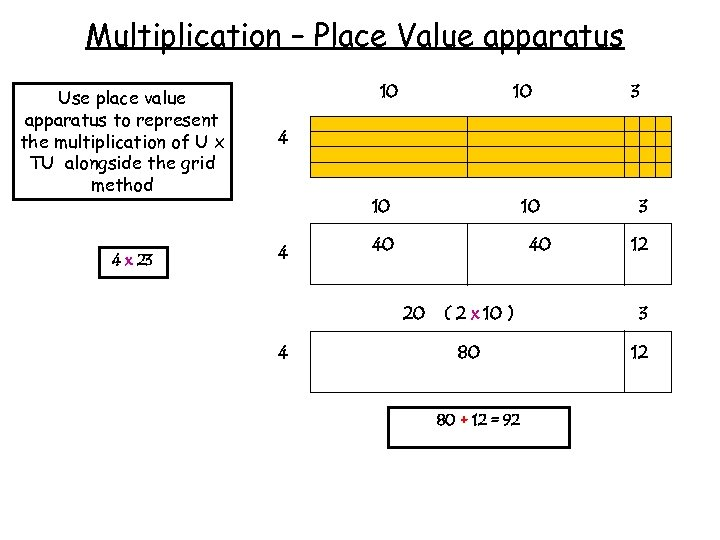Multiplication – Place Value apparatus Use place value apparatus to represent the multiplication of U x TU alongside the grid method 4 x 23 10 10 3 4 10 40 40 20 4 ( 2 x 10 ) 80 80 + 12 = 92 3 12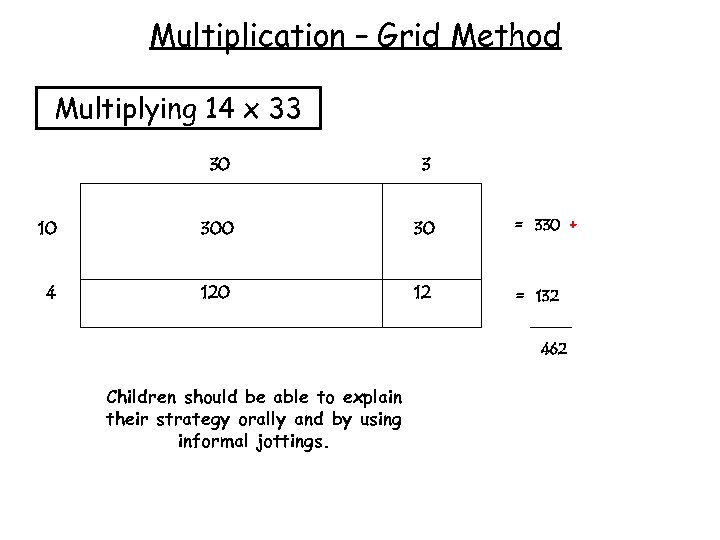Multiplication – Grid Method Multiplying 14 x 33 30 3 10 30 = 330 + 4 120 12 = 132 462 Children should be able to explain their strategy orally and by using informal jottings.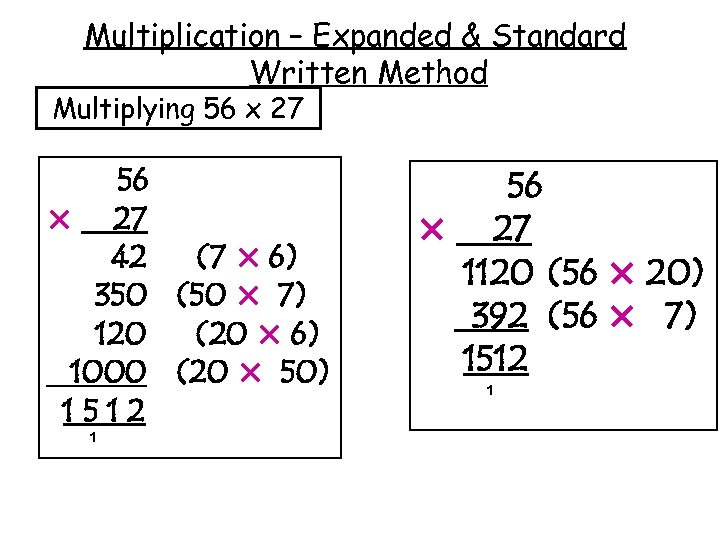Multiplication – Expanded & Standard Written Method Multiplying 56 x 27 56 × 27 42 (7 × 6) 350 (50 × 7) 120 (20 × 6) 1000 (20 × 50) 1512 1 56 × 27 1120 (56 × 20) 392 (56 × 7) 1512 1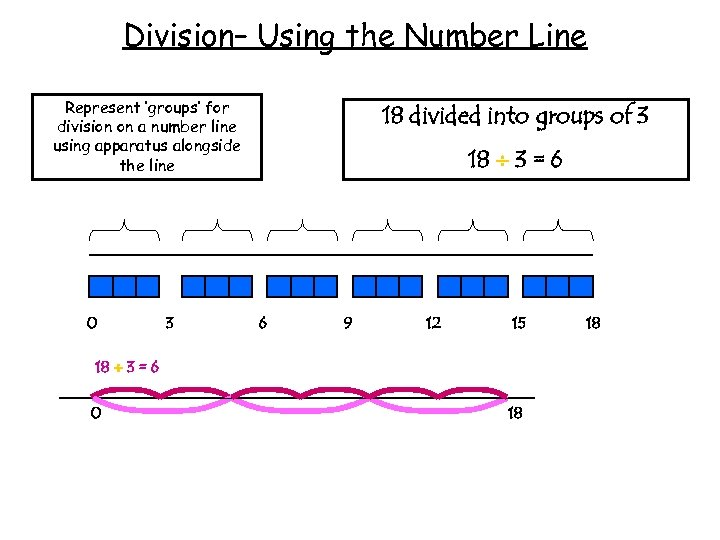Division– Using the Number Line Represent ‘groups’ for division on a number line using apparatus alongside the line 0 3 18 divided into groups of 3 18 3 = 6 6 9 12 15 18 3 = 6 0 18 18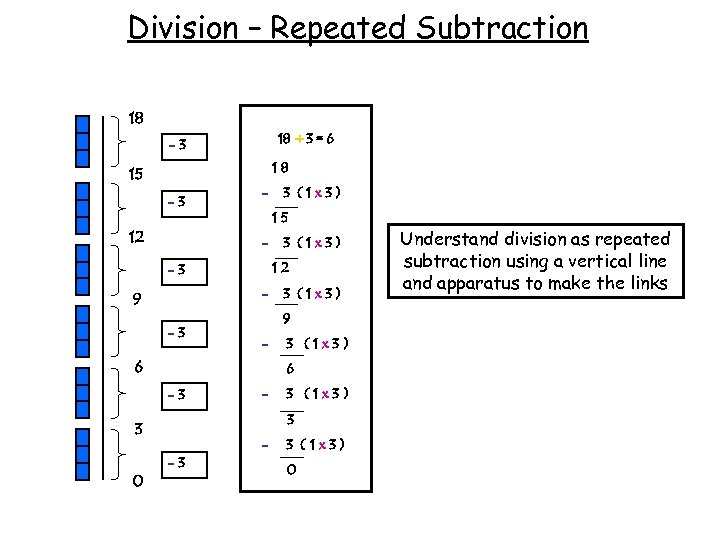Division – Repeated Subtraction 18 18 ÷ 3 = 6 -3 18 15 -3 12 - 15 - -3 3 (1 x 3) 9 - 6 3 (1 x 3) 6 -3 - 3 (1 x 3) 3 3 0 3 (1 x 3) 12 -3 9 3 (1 x 3) -3 3 (1 x 3) 0 Understand division as repeated subtraction using a vertical line and apparatus to make the links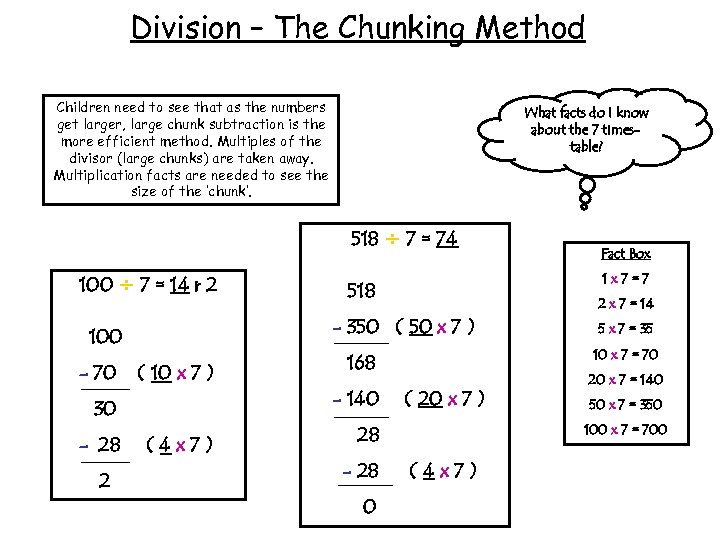Division – The Chunking Method Children need to see that as the numbers get larger, large chunk subtraction is the more efficient method. Multiples of the divisor (large chunks) are taken away. Multiplication facts are needed to see the size of the ‘chunk’. What facts do I know about the 7 timestable? 518 ÷ 7 = 74 100 ÷ 7 = 14 r 2 - 70 ( 10 x 7 ) 30 - 28 2 1 x 7=7 518 2 x 7 = 14 - 350 ( 50 x 7 ) 100 (4 x 7) ( 20 x 7 ) 0 20 x 7 = 140 50 x 7 = 350 100 x 7 = 700 28 - 28 5 x 7 = 35 10 x 7 = 70 168 - 140 Fact Box (4 x 7)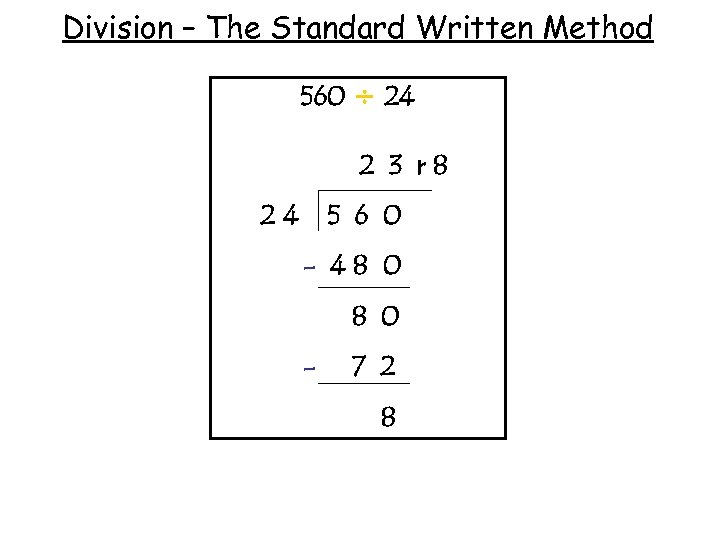Division – The Standard Written Method 560 ÷ 24 2 3 r 8 24 5 6 0 - 48 0 - 7 2 8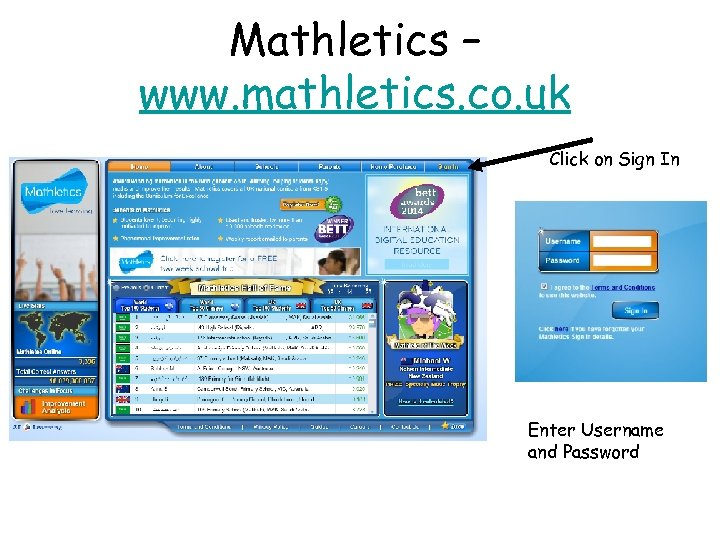Mathletics – www. mathletics. co. uk Click on Sign In Enter Username and Password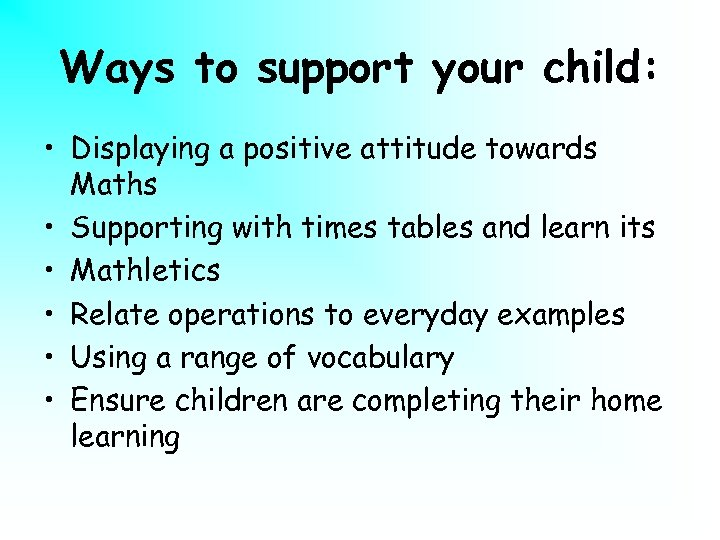Ways to support your child: • Displaying a positive attitude towards Maths • Supporting with times tables and learn its • Mathletics • Relate operations to everyday examples • Using a range of vocabulary • Ensure children are completing their home learning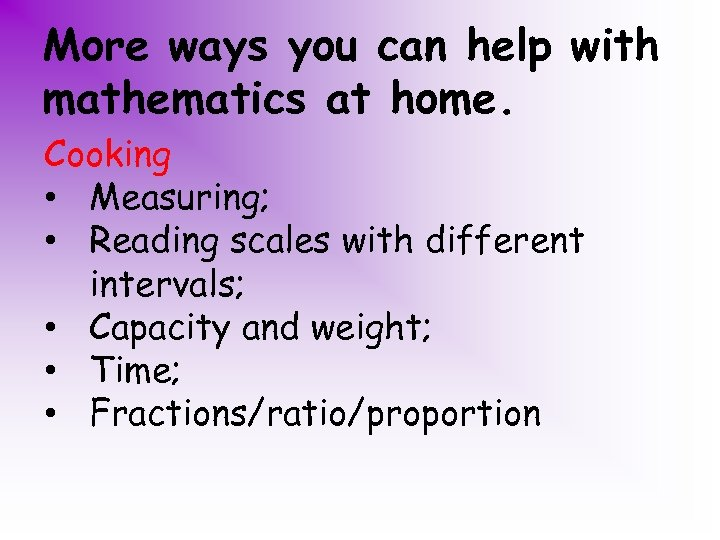More ways you can help with mathematics at home. Cooking • Measuring; • Reading scales with different intervals; • Capacity and weight; • Time; • Fractions/ratio/proportion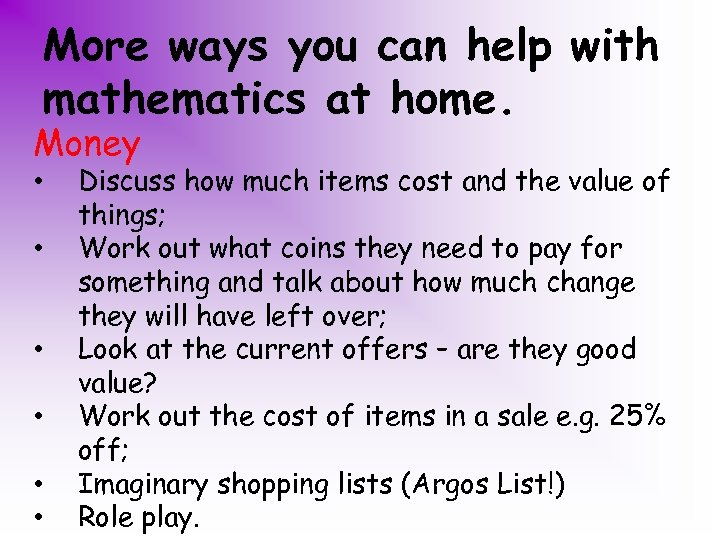More ways you can help with mathematics at home. Money • • • Discuss how much items cost and the value of things; Work out what coins they need to pay for something and talk about how much change they will have left over; Look at the current offers – are they good value? Work out the cost of items in a sale e. g. 25% off; Imaginary shopping lists (Argos List!) Role play.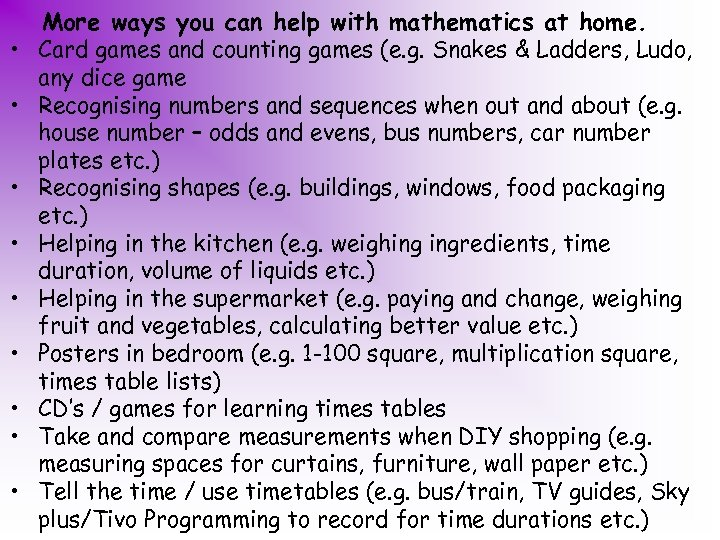• • • More ways you can help with mathematics at home. Card games and counting games (e. g. Snakes & Ladders, Ludo, any dice game Recognising numbers and sequences when out and about (e. g. house number – odds and evens, bus numbers, car number plates etc. ) Recognising shapes (e. g. buildings, windows, food packaging etc. ) Helping in the kitchen (e. g. weighing ingredients, time duration, volume of liquids etc. ) Helping in the supermarket (e. g. paying and change, weighing fruit and vegetables, calculating better value etc. ) Posters in bedroom (e. g. 1 -100 square, multiplication square, times table lists) CD’s / games for learning times tables Take and compare measurements when DIY shopping (e. g. measuring spaces for curtains, furniture, wall paper etc. ) Tell the time / use timetables (e. g. bus/train, TV guides, Sky plus/Tivo Programming to record for time durations etc. )Any Questions? Thank you for coming.